## Diffusion Coefficient

Fluid Flow, Heat Transfer, and Mass Transport   Diffusion   Diffusion Coefficient

### Understanding the Diffusion Coefficient

In dilute species transport, the flux due to diffusion is given by Fick's first law, which only depends on a single property of the solute's interaction with the solvent: the diffusion coefficient. The diffusion coefficient is most simply understood as the magnitude of the molar flux through a surface per unit concentration gradient out-of-plane. It is analogous to the property of thermal diffusivity in heat transfer:

(1)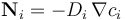so

(2)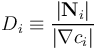A typical diffusion coefficient for a molecule in the gas phase is in the range of 10-6 to 10-5 m2/s. By contrast, diffusion for molecules dissolved in liquids is far slower. In an aqueous (water) solution, typical diffusion coefficients are in the range of 10-10 to 10-9 m2/s. As a result, diffusion in liquids is very slow over everyday length scales and is almost always dominated by convection.

From the above definition, the diffusion coefficient has units of area per time (m2 s-1, or m2/s in SI units). These units are also clear from a dimensional analysis of Fick's second law (also called the Diffusion equation). Formally, the diffusion coefficient can be understood as parameterizing the area of a spherical surface, defined as the surface of root-mean-square displacement of material diffusing away from an infinitesimal point where a mass is initially concentrated. Since the statistics of diffusion cause this area to grow linearly in time, the diffusion coefficient is a quantity described by area per time.

### Dependence on Other Properties

The diffusion coefficient can be predicted from first principles in some simple cases. By taking the values for the mean free path and average velocity for molecules in an ideal gas from the Maxwell-Boltzmann distribution, it follows that the diffusion coefficient obeys the following relation to temperature and pressure:

(3)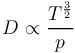That is, diffusion is faster in both hotter and more rarefied gases.

For particles or large molecules in a viscous fluid (usually a liquid solution), the Stokes-Einstein equation can be applied:

(4)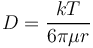Here, k is the Boltzmann constant, μ is the solvent viscosity, and r is the radius of the diffusing particle. This equation is derived on the assumption that the particles obey Stokes' law for drag, such that the drag exerted on diffusing molecules, by the solvent molecules, can be computed. Note that solvent viscosity itself strongly depends on temperature, so this equation does not imply a linear relation of solution-phase diffusion coefficient with temperature. Rather, the diffusion coefficient normally obeys a relation close to an exponential Arrhenius relation:

(5)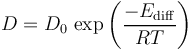Here, Ediff is an "activation energy of diffusion"; the exponential form of this relation means that diffusion coefficients in the solution phase can grow quickly with temperature.

### Diffusion Coefficient in Porous Media

In a porous medium, the effective diffusion coefficient becomes different from the real diffusion coefficient. This is because the available cross section for diffusion is less than for the free fluid and the distance between one point and another in the porous material is less than the distance that a molecule must travel to move between these points (since the molecule must navigate between the solid parts of the material). As a result, the real concentration gradient is less than the apparent concentration gradient. This effect is accounted for by multiplying the diffusion coefficient by a tortuosity (τ > 1) in Fick's first law:

(6)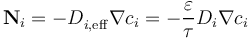Here, the fluxes and concentration gradient refer to areas and lengths measured with respect to the porous medium as a whole, rather than solely with respect to its fluid component in which diffusion can occur. The tortuosity is, then, the ratio of the actual distance a molecule must travel between two points by following the fluid channel to the straight-line distance between those points.

There are standard correlations relating the tortuosity to the porosity, ε:

Millington-Quirk:

(7)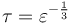Bruggeman:

(8)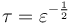In some types of porous media, the (effective) diffusion coefficient may also be anisotropic, so that the rate of diffusion depends on the direction of the concentration gradient. In this case, the diffusivity is a tensor.

### Multi-Component Diffusion

In concentrated mixtures, the effective diffusivity is a tensor where the mass flux of one species depends on the concentration gradients of all chemical species in the system. The diffusivities then reflect the interactions between each pair of species in a solution, also including the interaction between molecules of the same species.

Published: January 14, 2015